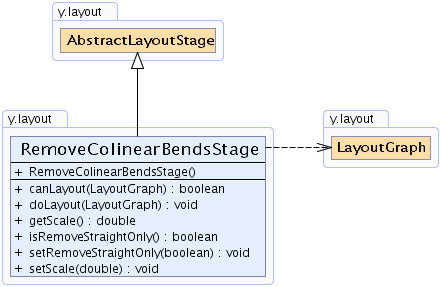Search this API

## y.layout Class RemoveColinearBendsStage

```java.lang.Objecty.layout.AbstractLayoutStagey.layout.RemoveColinearBendsStage
```
All Implemented Interfaces:
Layouter, LayoutStage

`public class RemoveColinearBendsStageextends AbstractLayoutStage`

The `RemoveColinearBendsStage` removes all collinear bends found in a graph. The algorithm traverses each edge path from source to target and removes for each triple of consecutive collinear bends the middle one.

A collinear bend forms a straight line with its preceding bend and its subsequent bend. The angle between the connecting edge segments is either `0` or `180` degrees.Field Summary

Fields inherited from interface y.layout.Layouter
`EDGE_ID_DPKEY, NODE_ID_DPKEY, SELECTED_EDGES, SELECTED_NODES`

Constructor Summary
`RemoveColinearBendsStage()`
Creates a new `RemoveColinearBendsStage` instance with default settings.

Method Summary
` boolean` `canLayout(LayoutGraph graph)`
Accepts all general graphs.
` void` `doLayout(LayoutGraph graph)`
Removes collinear bends of the edges in the given graph.
` double` `getScale()`
Returns the scale that defines how accurate the algorithm is when it comes to comparing the coordinates of two bends.
` boolean` `isRemoveStraightOnly()`
Returns whether the algorithm removes only collinear bends which lie in the middle of their neighbors or all collinear bends.
` void` `setRemoveStraightOnly(boolean removeStraightOnly)`
Specifies whether the algorithm removes only collinear bends which lie in the middle of their neighbors or all collinear bends.
` void` `setScale(double scale)`
Specifies the scale that defines how accurate the algorithm is when it comes to comparing the coordinates of two bends.

Methods inherited from class y.layout.AbstractLayoutStage
`canLayoutCore, doLayoutCore, getCoreLayouter, setCoreLayouter`

Methods inherited from class java.lang.Object
`clone, equals, finalize, getClass, hashCode, notify, notifyAll, toString, wait, wait, wait`

Constructor Detail

### RemoveColinearBendsStage

`public RemoveColinearBendsStage()`
Creates a new `RemoveColinearBendsStage` instance with default settings.

Method Detail

### canLayout

`public boolean canLayout(LayoutGraph graph)`
Accepts all general graphs.

Parameters:
`graph` - the input graph
Returns:
`true` for all graphs
`Layouter.doLayout(LayoutGraph)`

### getScale

`public double getScale()`
Returns the scale that defines how accurate the algorithm is when it comes to comparing the coordinates of two bends.

This value determines how the values of the coordinates are rounded. If rounded coordinates lead to a collinear bend, this bend will be removed. The larger the value, the higher the precision is.

• Positive scale values: the value denotes the number of decimal positions that are considered (e.g. `10.2345` becomes `10.23` for a scale factor of `2`).
• Scale value zero: coordinates are rounded to the next integer coordinates (e.g. `10.2345` becomes `10`).
• Negative scale values: the coordinates are rounded to the next multiple of `10`, `100` and so on (e.g. `10.2345` becomes `0` for a scale factor of `-2`).

Returns:
the scale
`setScale(double)`

### setScale

`public void setScale(double scale)`
Specifies the scale that defines how accurate the algorithm is when it comes to comparing the coordinates of two bends.

This value determines how the values of the coordinates are rounded. If rounded coordinates lead to a collinear bend, this bend will be removed. The larger the value, the higher the precision is.

• Positive scale values: the value denotes the number of decimal positions that are considered (e.g. `10.2345` becomes `10.23` for a scale factor of `2`).
• Scale value zero: coordinates are rounded to the next integer coordinates (e.g. `10.2345` becomes `10`).
• Negative scale values: the coordinates are rounded to the next multiple of `10`, `100` and so on (e.g. `10.2345` becomes `0` for a scale factor of `-2`).

Default Value:
The default value is 2. Bend coordinates are rounded to the second decimal position before comparing.
Parameters:
`scale` - the scale

### isRemoveStraightOnly

`public boolean isRemoveStraightOnly()`
Returns whether the algorithm removes only collinear bends which lie in the middle of their neighbors or all collinear bends.

In the first case, the angle at the middle bend must be `180` degrees, while in the second case, the angle can be either `180` degrees or `0` degrees.

Returns:
`true` if only bends with `180` degrees are removed, `false` otherwise

### setRemoveStraightOnly

`public void setRemoveStraightOnly(boolean removeStraightOnly)`
Specifies whether the algorithm removes only collinear bends which lie in the middle of their neighbors or all collinear bends.

In the first case, the angle at the middle bend must be `180` degrees, while in the second case, the angle can be either `180` degrees or `0` degrees.

Default Value:
The default value is false. All collinear bends are removed from the edges.
Parameters:
`removeStraightOnly` - `true` if only bends with `180` degrees are removed, `false` otherwise

### doLayout

`public void doLayout(LayoutGraph graph)`
Removes collinear bends of the edges in the given graph.

Parameters:
`graph` - the input graph
`Layouter.canLayout(LayoutGraph)`